# Merton model

Financial institutions like banks and insurances companies, need to assess the credit risk of their loan and bond portfolio and individual holdings. One way to do this by using a structural credit risk model. This is a kind of model that imposes a structure on certain economic indicators or financial ratios. These indicators may explain the credit risk and probability of default of a certain company. For example, companies with higher debt ratios may be tied to higher defaults and thus credit risk.

## Merton credit risk model

Merton developed a structural model based on the Black Scholes option pricing model. This model  can both be used for equity valuation and credit risk management. The intuition behind the use of option pricing for equity valuation in the Merton model is simple. Equity holders are the residual owners of a company. The value of the assets above debt K, will be paid out to them. Therefore, Merton argues that equity holders have a call option on the company’s assets with strike price K. If the value of assets is larger then K, then the get V– K. If not, then they get nothing. In contrast, the bondholders are argued to own a zero-coupon bond with par value of K. They retrieve the value of K when the assets are worth more. If the assets are worth less, then they receive only the asset value VT. Bondholders are thus short a put option.

## Merton model formula & distance to default

The formula below values the equity in function of the value of assets corrected for the value of debt. The additional parameters required are the risk free rate, r, the volatility of assets, σv, and the time to maturity T. The higher the value of assets is, and the lower the debt, the more valuable equity is.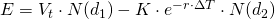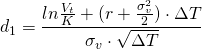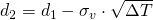The problem now is that the value of assets is not observable, nor is the volatility of assets. What is observable is the value of equity and it volatility in case the company is listed. Additionally, σE and σV are related as follows. With this additional equation, the value of the asses, V, and the volatility of assets, σV, can be iteratively estimated as shown in the provided Excel spreadsheet below.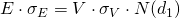## What is probability of default?

The Merton model also allows to estimate a probability of default. This indicates the chance that a company will not be able to repay its debt considering a certain time frame, in many cases 1 year. The Merton model allows to calculate a risk-neutral probability of default for a certain company. The risk neutral PD indicates the chance that at maturity the value of assets will be worth less than the debt. Just like for option, it indicates the probability that the option ends up out-of-the money. The risk neutral probability of default is calculated as follows.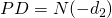## Summary

The Merton model assesses the value of equity for stock listed companies based on option pricing theory. Additionally, it can be used to estimate risk neutral probabilities of default which can be used for credit risk management purposes

### Merton model

Need to have more insights? Download our free excel file: merton model.